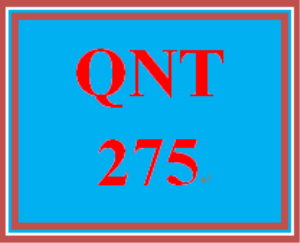# QNT 275 Week 3 Apply: Connect Week 3 Case

PLDZ-7498 In Stock
\$ 12.99 USD
Description

https://uopcourses.com/category/qnt-275/

More courses: https://uopcourses.com/

QNT 275 Week 3 Apply: Connect Week 3 Case

Part 1
Three hundred consumers between 21 and 49 years old were randomly selected. After sampling a new wine cooler, each was asked to rate the appeal of the phrase: "Not sweet like wine coolers, not filling like beer, and more refreshing than wine or mixed drinks" as it relates to the new wine cooler. The rating was made on a scale from 1 to 5, with 5 representing "extremely appealing" and with 1 representing "not at all appealing".

As a manager overseeing the development of the concept, you bottle the wine cooler and placed it into distribution in one test store.

Your manager has asked you to assess the data and determine the most likely customer based on the ratings. Additionally, your manager would like you to review sales in the test store.

Use the Week 3 Data Set to create and calculate the following in Excel®:

Estimate the probability that a randomly selected 21 to 49 year old consumer:
Would give the phrase a rating of 5
Would give the phrase a rating of 3 or higher
Is in the 21-24 age group
Is a male who gives the phrase a rating of 4
Is a 35 to 49 year old who gives the phrase a rating of 1
Based on the probabilities for the ratings of 4 and 5, which age/gender demographic would be the best target audience for the new concept?
Create a probability distribution using the data which shows how many cartons of the wine cooler were bought per customer in a month.
Calculate the mean and the standard deviation of your probability distribution.
Calculate the probability that exactly 3 six packs will be bought in a month.
Calculate the probability that between 4 and 8 six packs will be bought in a month.
Calculate the probability that at least 5 six packs will be bought in a month.
Calculate the probability that no more than 5 six packs will be bought in a month.
Create a relative frequency distribution based on the wine cooler drinking temperatures.
Create 6 bins with the same interval in each.
Create a histogram
Considering the mean and standard deviation for the ideal drinking temperature:
Calculate z values then refer to Table 6.1 - Cumulative Areas Under the Standard Normal Curve
Calculate the probability of the wine cooler being less than 45 degrees.
Calculate the probability of the wine cooler being greater than 60 degrees.
Calculate the percentage of wine coolers served at the ideal temperature, between 49 and 55 degrees.
Part 2